# How to make a Linear Regressor? (theory)

This post presents the formulas for coming up with the best-fitted linear regression line. If you want to practically make a regressor, read the post about How to make a Linear Regressor in Python.

Prerequisites: some Linear Algebra and Calculus are required to follow this post.

There are essentially 2 ways to make a Linear regressor from given training data and a cost function. Those 2 are:

• Closed-form Linear regression algorithm
• Gradient descent for Linear regression

For the cost function, we will use the Least Squared Error (LSE) in this post. The cost function’s formula is: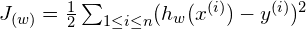where:

• w: the coefficients (the weights) in Linear regressor,
•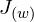: the cost of the regressor,
• n: the size of training data,
•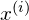: the i-th training data,
•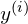: the true response-value of the i-th training data,
•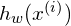: the predicted-value for the i-th training data,
•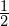: a constant added for mathematical convenience.

Note that the Linear regression that uses this cost function is also called an Ordinary Least Squares (OLS) regression.

1. Closed-form Linear Regression algorithm

Caveat: This closed-form algorithm of Linear Regression is only applicable if the cost function is LSE. For other cost functions, we should use the Gradient Descent approach.

The main idea behind this method is to minimize the cost function (J), we find its derivatives with respect to w and set this value to 0 , which is: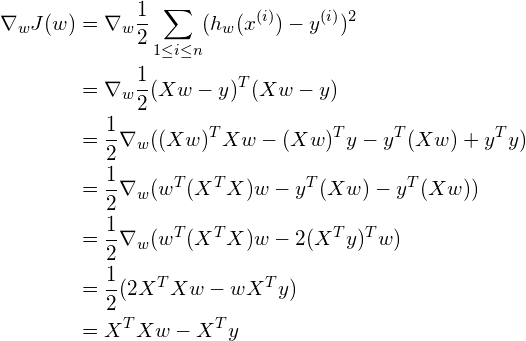where X is the training data.

In order to minimize J(w), we set its derivatives to 0, thus: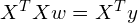which gives:

w =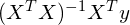Here we have the best-fitted line for the given data!

Unlike the Closed-form solution which can only work for LSE cost function, the Gradient Descent method can be applied for all cost functions, as long as they are differentiable.

If you haven’t heard about Gradient Descent, I suggest taking some time to read tutorials about it, as Gradient Descent is the basic technique for many optimization problems that we will encounter frequently.

In simple words, Gradient Descent (or Accent) is a greedy-like, iterative method that simulates the process of mountain climbing. Imagine optimizing the objective function is like climbing a mountain filled with fog, the higher position you can get to, the better your objective function is (or say the lower your cost function). Because of the fog, you cannot see far away, but only a radius of 1 meter around you is visible. At each step of the iteration, you look around to choose which direction to go next. As Gradient Descent is a greedy algorithm, you choose the local-best choice – the direction that gets you to the highest position in the 1-meter-radius around.

More specifically, the mountain climbing process is a metaphor for Gradient Descent as below:

For simplicity, in the following, we examine 1 sample at a time.

Let’s call d the number of predictor variables. Our regressor is consisting of d+1 parameters (one for each predictor variable and a constant). The Gradient Descent method works as:

Loop until converge:
For each sample data i (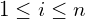):
Simultaneously for each j (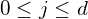):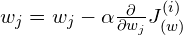where:

•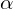being the Learning rate, which adjusts the intensity of our modification at each step.
•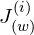is the cost (error) of i-th sample.

We can simplify the formula with: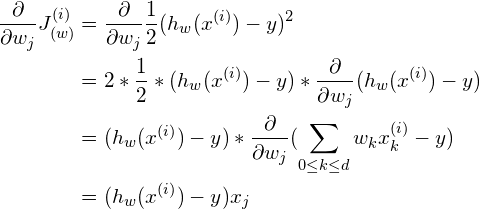More generally,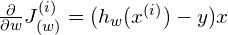In short, this is how we iteratively update the regressor’s parameters:

Loop until converge:
For each sample data i ():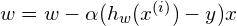It is so beautiful that after math transformations, the formula becomes very intuitive. We adjust the regressor’s parameters directly by the difference between predicted value and the true value.

In general, Gradient Descent, like many other optimization algorithms, has problems with struggling around local minima. However, in this case, our cost function (LSE) is convex and has only 1 global minimum, no local minimum, hence the algorithm always converges to the best possible result.

Practically, to reduce the number of updates on the regressor’s weights (to make training faster), at each step, we can examine a batch of samples instead of 1 sample alone (Mini-batch Gradient Descent). These batches can be mutually exclusive or just randomly chosen (Stochastic Gradient Descent).

Conclusion

Above, we walked through 2 methods to derive the best-fitted regressor from input data.

• The Closed-form formula gives a straight-forward recipe to make the regressor using Matrix multiplication. Note that this only works for LSE cost function.
• Gradient Descent is an iterative approach to this problem. This optimization algorithm can be used with any differentiable cost function, but can only guarantee to produce the optimal solution if the cost function is convex (e.g. LSE).

You can find the full series of blogs on Linear regression here.

References:

• Andrew Ng’s Machine Learning course CS229, note 1: link